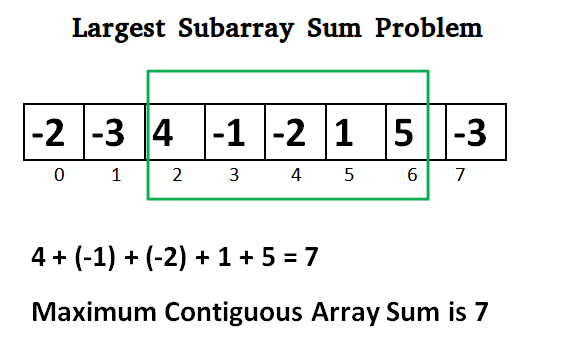Related Articles

# C# Program for Largest Sum Contiguous Subarray

• Last Updated : 03 Dec, 2019

Write an efficient program to find the sum of contiguous subarray within a one-dimensional array of numbers which has the largest sum.## C#

 `// C# program to print largest``// contiguous array sum``using` `System;`` ` `class` `GFG {``    ``static` `int` `maxSubArraySum(``int``[] a)``    ``{``        ``int` `size = a.Length;``        ``int` `max_so_far = ``int``.MinValue,``            ``max_ending_here = 0;`` ` `        ``for` `(``int` `i = 0; i < size; i++) {``            ``max_ending_here = max_ending_here + a[i];`` ` `            ``if` `(max_so_far < max_ending_here)``                ``max_so_far = max_ending_here;`` ` `            ``if` `(max_ending_here < 0)``                ``max_ending_here = 0;``        ``}`` ` `        ``return` `max_so_far;``    ``}`` ` `    ``// Driver code``    ``public` `static` `void` `Main()``    ``{``        ``int``[] a = { -2, -3, 4, -1, -2, 1, 5, -3 };``        ``Console.Write(``"Maximum contiguous sum is "` `+ maxSubArraySum(a));``    ``}``}`` ` `// This code is contributed by Sam007_`
Output:
```Maximum contiguous sum is 7
```

Above program can be optimized further, if we compare max_so_far with max_ending_here only if max_ending_here is greater than 0.

## C#

 `static` `int` `maxSubArraySum(``int``[] a,``                          ``int` `size)``{``    ``int` `max_so_far = 0,``        ``max_ending_here = 0;`` ` `    ``for` `(``int` `i = 0; i < size; i++) {``        ``max_ending_here = max_ending_here + a[i];``        ``if` `(max_ending_here < 0)``            ``max_ending_here = 0;`` ` `        ``/* Do not compare for all``    ``elements. Compare only ``    ``when max_ending_here > 0 */``        ``else` `if` `(max_so_far < max_ending_here)``            ``max_so_far = max_ending_here;``    ``}``    ``return` `max_so_far;``}`` ` `// This code is contributed``// by ChitraNayal`

Please refer complete article on Largest Sum Contiguous Subarray for more details!

My Personal Notes arrow_drop_up